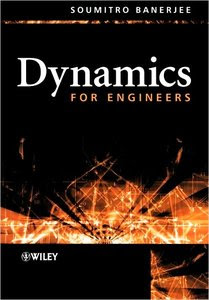Telugu and Hindi movies are posted on request!! Post your requests to TELUGUSINEMA@GMAIL.COM

Dynamics for EngineersModelling and analysis of dynamical systems is a widespread practice as it is important for engineers to know how a given physical or engineering system will behave under specific circumstances.
This text provides a comprehensive and systematic introduction to the methods and techniques used for translating physical problems into mathematical language, focusing on both linear and nonlinear systems. Highly practical in its approach, with solved examples, summaries, and sets of problems for each chapter, Dynamics for Engineers covers all aspects of the modelling and analysis of dynamical systems.
Key features:
* Introduces the Newtonian, Lagrangian, Hamiltonian, and Bond Graph methodologies, and illustrates how these can be effectively used for obtaining differential equations for a wide variety of mechanical, electrical, and electromechanical systems.
* Develops a geometric understanding of the dynamics of physical systems by introducing the state space, and the character of the vector field around equilibrium points.
* Sets out features of the dynamics of nonlinear systems, such as like limit cycles, high-period orbits, and chaotic orbits.
* Establishes methodologies for formulating discrete-time models, and for developing dynamics in discrete state space.
Senior undergraduate and graduate students in electrical, mechanical, civil, aeronautical and allied branches of engineering will find this book a valuable resource, as will lecturers in system modelling, analysis, control and design. This text will also be useful for students and engineers in the field of mechatronics.